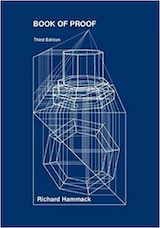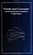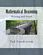Processing ......FreeComputerBooks.com Links to Free Computer, Mathematics, Technical Books all over the World

Book of Proof, Third Edition
🌠 Top Free Unix/Linux Books - 100% Free or Open Source!
• Title Book of Proof, Third Edition
• Author(s) Richard Heath Hammack
• Publisher: Richard Hammack, Third Edition (2018); eBook (Creative Commons Licensed)
• Paperback 312 pages
• eBook PDF Files
• Language: English
• ISBN-10: 0989472124, 0989472108 (2nd edition)
• ISBN-13: 978-0989472128, 978-0989472104 (2nd edition)Book Description

This book is an introduction to the language and standard proof methods of mathematics.

It is a bridge from the computational courses (such as calculus or differential equations) that students typically encounter in their first year of college to a more abstract outlook. It lays a foundation for more theoretical courses such as topology, analysis and abstract algebra. Although it may be more meaningful to the student who has had some calculus, there is really no prerequisite other than a measure of mathematical maturity.

Topics include sets, logic, counting, methods of conditional and non-conditional proof, disproof, induction, relations, functions, calculus proofs and infinite cardinality.

• Richard Hammack is a professor of mathematics at Virginia Commonwealth University in Richmond, Virginia. A native of rural southern Virginia, he studied painting at Rhode Island School of Design before an interest in computer graphics and visualization led him to mathematics. He works mostly in the areas of combinatorics and graph theory.
Reviews, Ratings, and Recommendations: Related Book Categories: Read and Download Links:Similar Books:
•Proof, Sets, and Logic (M. Randall Holmes)

Addressing the importance of constructing and understanding mathematical proofs, this book introduces key concepts from logic and set theory as well as the fundamental definitions of algebra to prepare readers for further study in the field of mathematics.

•Proofs and Concepts: The Fundamentals of Abstract Mathematics

This undergraduate textbook provides an introduction to proofs, logic, sets, functions, and other fundamental topics of abstract mathematics. It helps students transition from solving problems to proving theorems.

•Mathematical Reasoning: Writing and Proof (Ted Sundstrom)

Help students to develop logical thinking skills and to think abstractly, and write mathematical proofs using standard methods of mathematical proof including direct proofs, proof by contradiction, mathematical induction, case analysis, counterexamples.

•Language, Proof and Logic (Jon Barwise, et al)

This book covers first-order language in a method appropriate for first and second courses in logic, and is specially useful to undergraduates of philosophy, computer science, mathematics, and linguistics.

•An Introduction to Mathematical Reasoning (Peter J. Eccles)

The purpose of this book is to introduce the basic ideas of mathematical proof to students embarking on university mathematics. It eases students into the rigors of university mathematics.

•Logical Reasoning (Bradley H. Dowden)

The goal of this book is to improve your logical-reasoning skills. Your most important critical thinking skill is your skill at making judgments-not snap judgments that occur in the blink of an eye, but those that require careful reasoning.

•Math Alive (Ingrid Daubechies, et at)

Mathematics has profoundly changed our world, from banking to listening to music. This course is designed for those who haven't had college mathematics but would like to understand some of the mathematical concepts behind important modern applications.

Book Categories
 :All CategoriesTop Free BooksRecent BooksMiscellaneous BooksComputer EngineeringComputer LanguagesComputer ScienceData Science/DatabasesJava and Java EE (J2EE)Linux and UnixMathematicsMicrosoft and .NETMobile ComputingNetworking and CommunicationsSoftware EngineeringSpecial TopicsWeb Programming
Other Categories